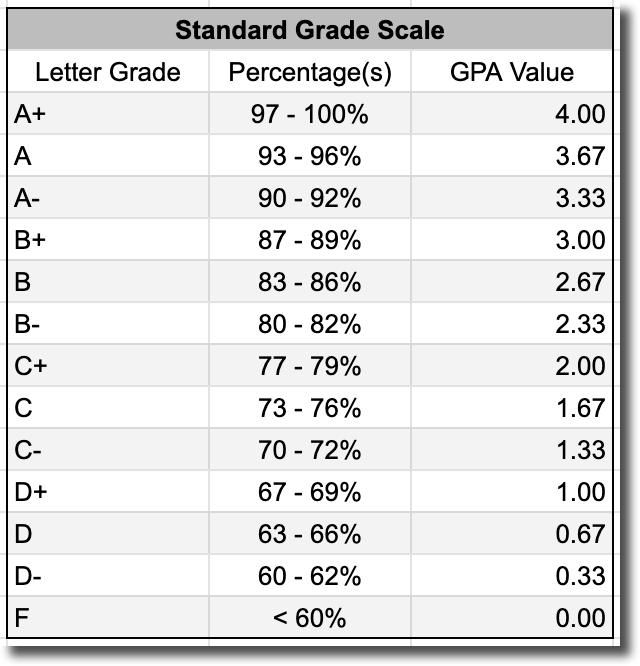Scenario:

2. We believe that Gradelink is not calculating our GPA correctly.

Solution:

In Gradelink, GPA is calculated via the following method:

1. Take the class grades achieved by a student and convert them into a "GPA value".
2. Multiply the "GPA values" by the number of units/credits each respective class is worth.
3. Add the new "GPA values" together.
4. Divide the total "GPA value" by the total number of units/credits attempted.

You can see this method in action in the example below.You may be wondering, why doesn't Gradelink average the GPA values from each class?

In the example shown above, averaging the GPA Value for each class produces a GPA of 3.67, which is higher than the 3.63 that we calculated.

The reason Gradelink doesn't simply average the GPA values is because doing so implies that each class has the same amount of influence on the final grade. In the example above, the "Physical Education" class is only worth 2 units, so if we average the GPA values together and ignore the unit count, the end result is that each class becomes worth the same amount to the overall GPA.

If we calculate the GPA with the "Physical Education" class worth 5 units instead of 2, we will still arrive at a GPA of 3.67, so adding this extra step to the process doesn't change anything as long as the units/credits for each class are configured correctly.Multiplying the GPA value of each class by the number of units that class is worth ensures that each grade contributes to the GPA in the correct proportion.